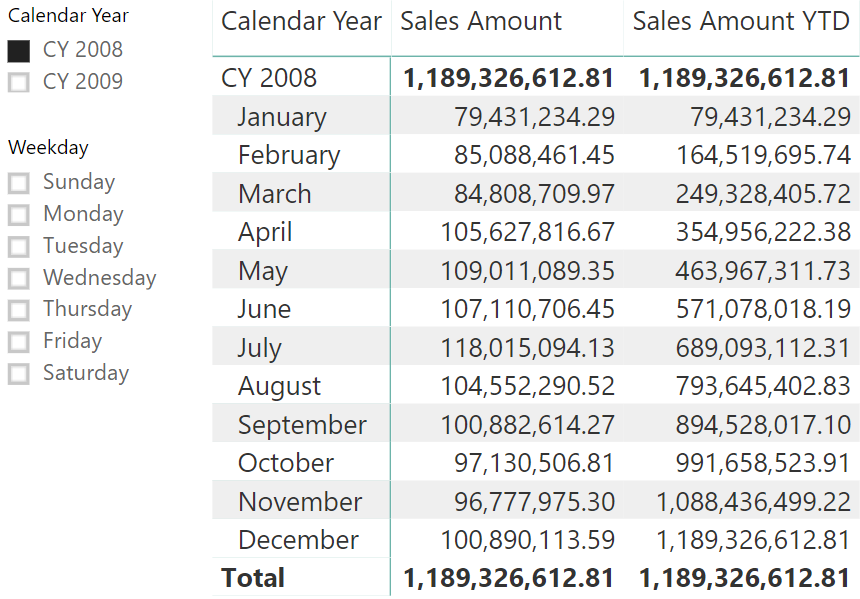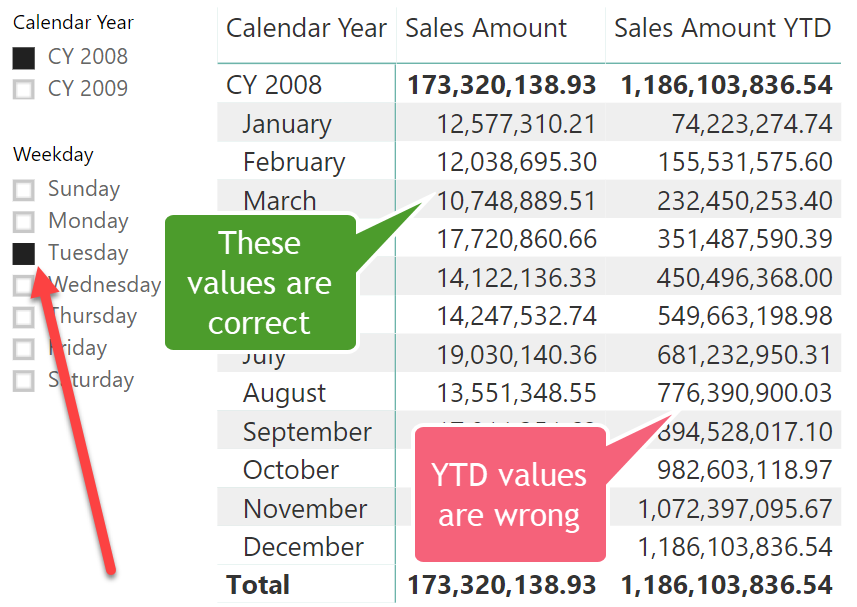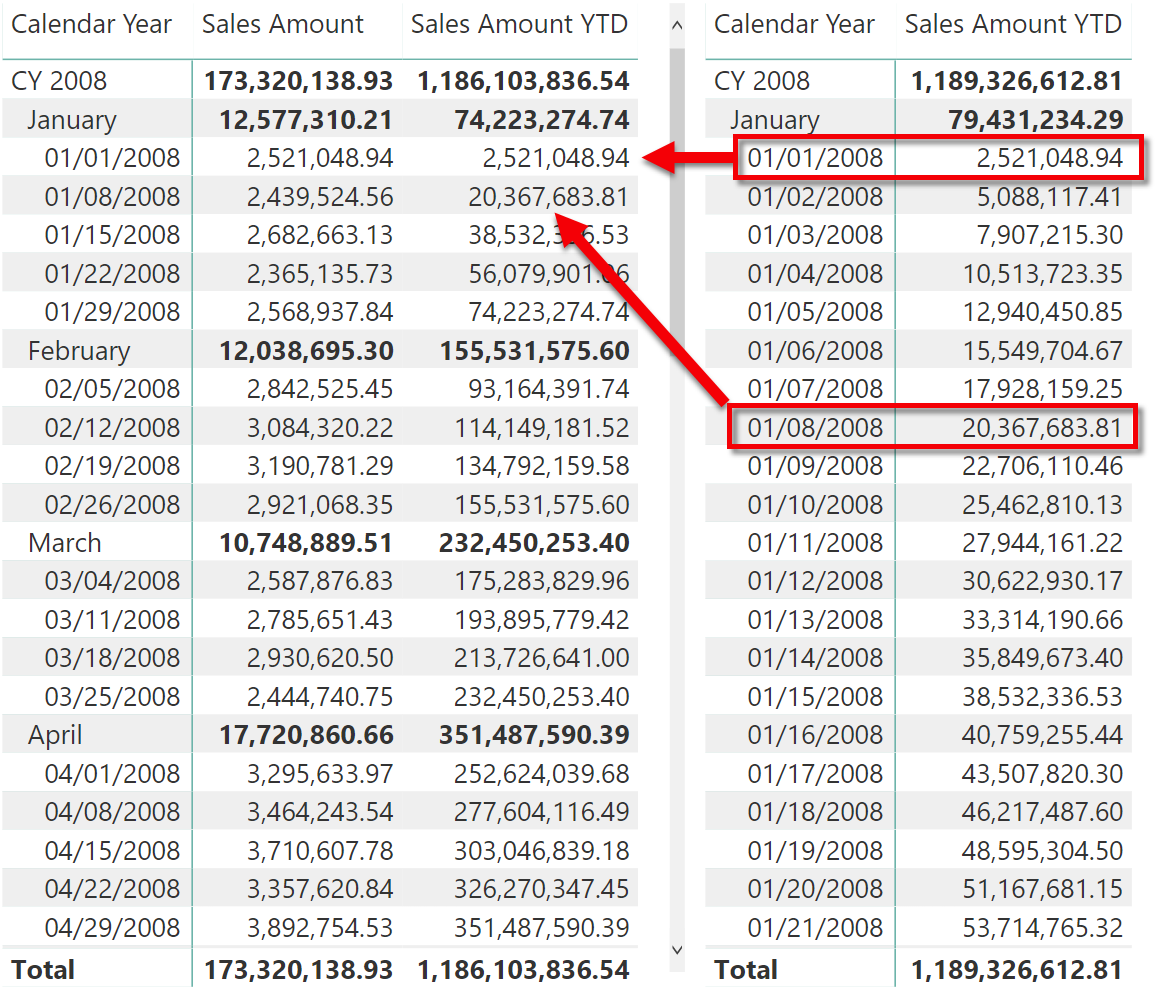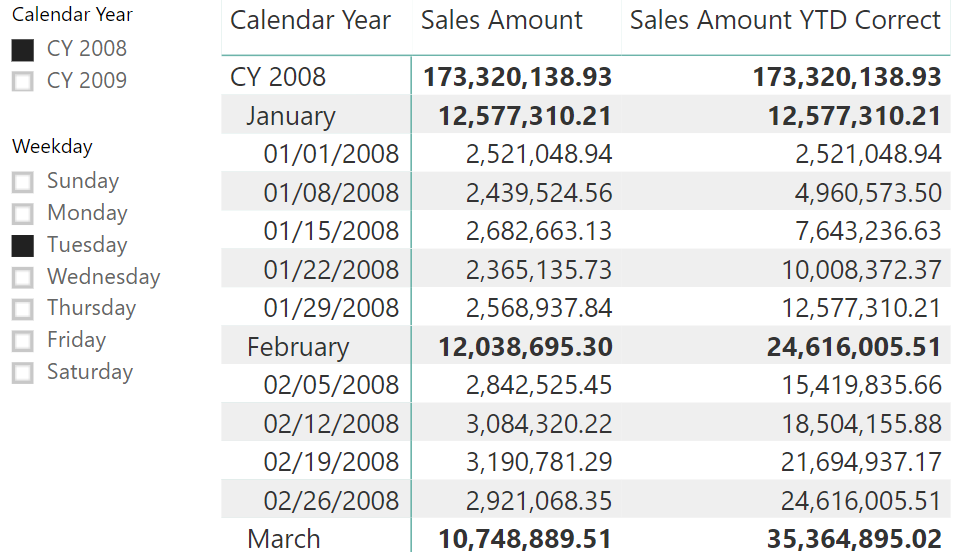UPDATE 2020-11-10: You can find more complete detailed and optimized examples for the following scenario in the Time patterns articles+videos on daxpatterns.com.

Consider a simple measure that computes year-to-date (YTD) sales amounts:

```Sales Amount YTD :=
CALCULATE (
[Sales Amount],
DATESYTD ( 'Date'[Date] )
)
```

Used in a simple matrix, the measure produces the expected result:It is worth noting that the slicer filtering by Year is working as expected – the matrix only shows data in CY 2008, excluding data in CY 2009.

Surprisingly, the slicer filtering the weekday does not produce the same result, as it appears in the following report:At first sight, the numbers displayed for the YTD calculation make no sense at all. It is thus helpful to look at the previous report side-by-side with a non-filtered regular YTD calculation:As expected, the filtered matrix only displays Tuesdays. However, instead of being the cumulative sum of Tuesdays exclusively, the value is simply extracted from whatever the overall YTD value happens to be on those Tuesdays. Furthermore, in the figure above, at the month level the value shown in bold is the overall YTD as calculated on the last Tuesday of the month. This is definitely counterintuitive.

The reason is that the relationship between the fact table and the dimension is based on a column which has a Datetime data type. Whenever this is the case, DAX automatically adds an ALL statement on the whole table when you use a CALCULATE to modify the filter on the date. The same behavior happens if the table is marked as a Date table, using the feature available since the February 2018 update of Power BI.

If DAX did not behave this way, then the regular YTD calculation would not work. In fact, in every cell of the regular YTD, the filters on month and year would still be active and DATESYTD would only add a filter instead of replacing the current filter. This behavior of time intelligence calculations is intuitive and makes it easy to author most of the time intelligence functionalities. However, in some specific cases like the one analyzed in this article, it will create the issue described above.

Once the problem becomes clear, the solution is straightforward: the formula needs to take into account the existing filter context on the weekday and, because the hidden ALL removes that existing filter context, adding it again in CALCULATE does the trick:

```Sales Amount YTD Correct =
CALCULATE (
[Sales Amount],
DATESYTD( 'Date'[Date] ),
VALUES ( 'Date'[Weekday] )
)
```

Now, as expected, the result is a YTD considering only Tuesdays:This technique works for calculations that simply aggregates data, like year-to-date and quarter-to-date. In case you have this issue for comparing data in different periods (for example using SAMEPERIODLASTYEAR), then the solution is to get rid of time intelligence functions, writing a complete custom filter logic that satisfies specific requirements – which could be the topic for a future article.

UPDATE 2020-09-04: The section “Filtering other date attributes” in the Standard time-related calculations pattern describes the pattern to use to implement the technique described in this article.

ALLCALCULATE modifier

Returns all the rows in a table, or all the values in a column, ignoring any filters that might have been applied.

`ALL ( [<TableNameOrColumnName>] [, <ColumnName> [, <ColumnName> [, … ] ] ] )`

CALCULATEContext transition

Evaluates an expression in a context modified by filters.

`CALCULATE ( <Expression> [, <Filter> [, <Filter> [, … ] ] ] )`

DATESYTDContext transition

Returns a set of dates in the year up to the last date visible in the filter context.

`DATESYTD ( <Dates> [, <YearEndDate>] )`

SAMEPERIODLASTYEARContext transition

Returns a set of dates in the current selection from the previous year.

`SAMEPERIODLASTYEAR ( <Dates> )`